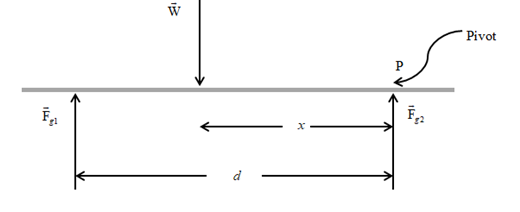Chapter 8, Problem 21P

Chapter
Section
Textbook ProblemTo determine
The location of the center of gravity from the woman’s feet.

Explanation

The following figure shows the forces on the woman.

Given info: The scale reading are 380N and 320N respectively, weight of the woman is 700N , the force acts on her head is 380N , and the separation between the forces Fg1 and Fg2 is 2.00m .

Explanation: Assume that the weight of the plank was neglected and the condition for the equilibrium is used and it could be written as Fy=0=Fg1+Fg2W . Consider the point where the force Fg2 acts as a pivot point and the condition of static torque on her about the point is defined as τ=0=xWdFg1 .

The formula for the weight of the woman is,

W=Fg1+Fg2

• Fg1 is the left scale reading.
• Fg2 is the right scale reading.

Substitute 380N for Fg1 and 320N for Fg2 to find W

Still sussing out bartleby?

Check out a sample textbook solution.

See a sample solution

The Solution to Your Study Problems

Bartleby provides explanations to thousands of textbook problems written by our experts, many with advanced degrees!

Get Started